# NCERT Exemplar Class 8 Maths Solutions for Chapter 5 - Understanding Quadrilaterals Practical Geometry

## NCERT Exemplar Solutions Class 8 Maths Chapter 5 – Free PDF Download

The NCERT Exemplar Class 8 Maths Chapter 5 Understanding Quadrilaterals & Practical Geometry is provided here for students to prepare for exams. These exemplars problems and solutions are designed by experts in accordance with the CBSE syllabus (2023-2024) for the 8th standard, which covers all the topics of Maths Chapter 5. The chapter – Understanding Quadrilaterals and Practical Geometry – is about learning the basics of quadrilaterals and geometry. The first part of understanding quadrilaterals is about learning about polygons, parallelograms and the properties of parallelograms. In the second part, the students will learn how to construct geometrical figures like quadrilaterals and triangles. To understand the concepts and score good marks in the final exam, students are advised to solve questions from the NCERT Maths Exemplar for Class 8.

Solving Exemplar Problems for Class 8 will help students to understand the concepts present in each chapter of Maths as well as Science subjects. Below is the list of topics covered in Chapter 5 of Class 8 Maths.

• Polygons such as triangles, quadrilaterals, pentagons, etc.
• Diagonals of polygons, convex and concave polygons
• Regular and irregular polygons and angle sum property for polygons
• Learn to construct a quadrilateral depending on the measurements

Apart from these exemplars, BYJU’S provides notes, exemplar books, Maths NCERT Solutions for 8th standard and question papers to help students practise well for exams. Also, it is advised for learners to solve sample papers and previous years’ question papers, which gives an idea of the types of questions asked in the board exam from Understanding Quadrilaterals & Practical Geometry.

### NCERT Exemplar Class 8 Maths Solutions Chapter 5 Understanding Quadrilaterals Practical Geometry:-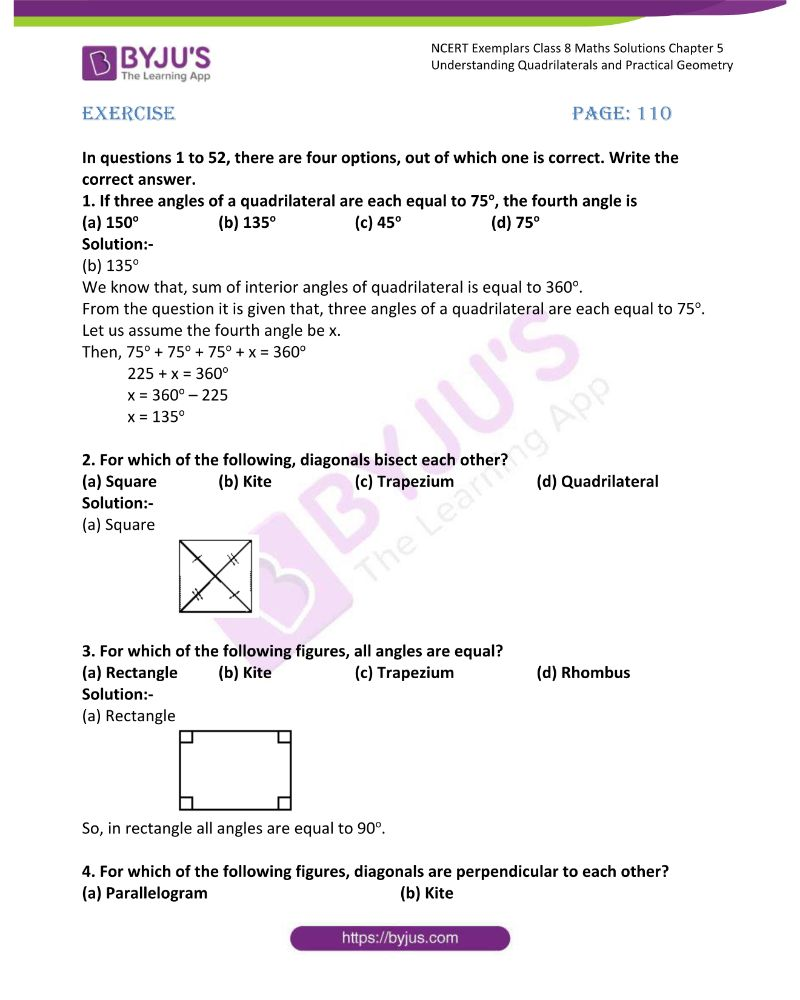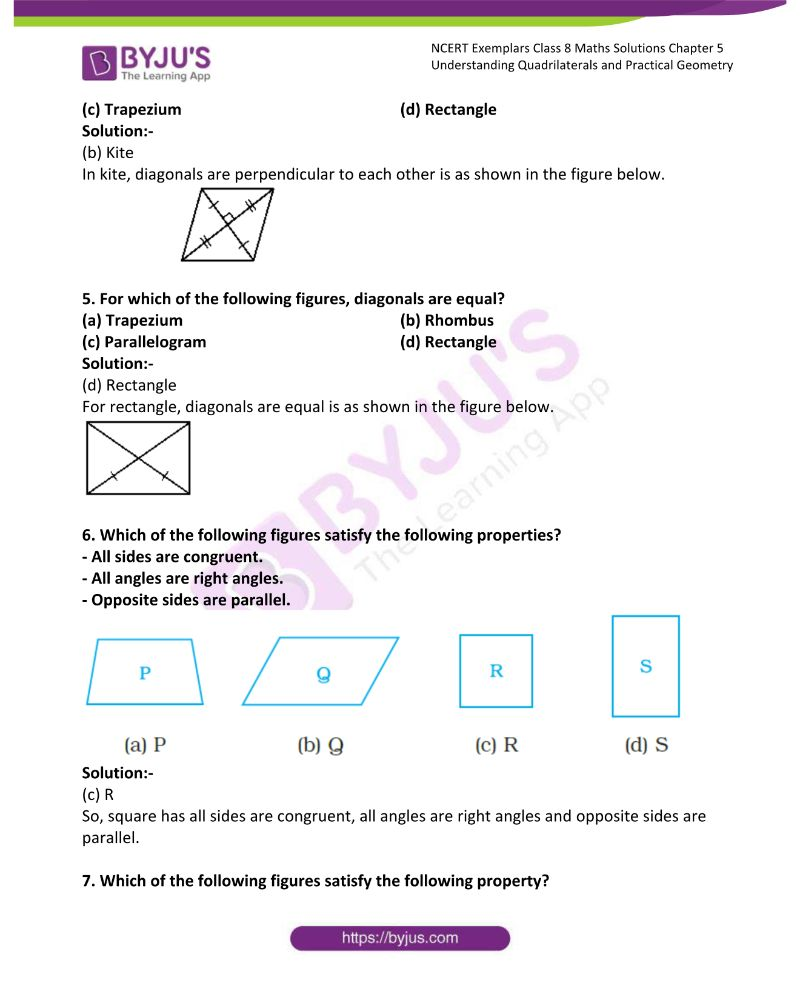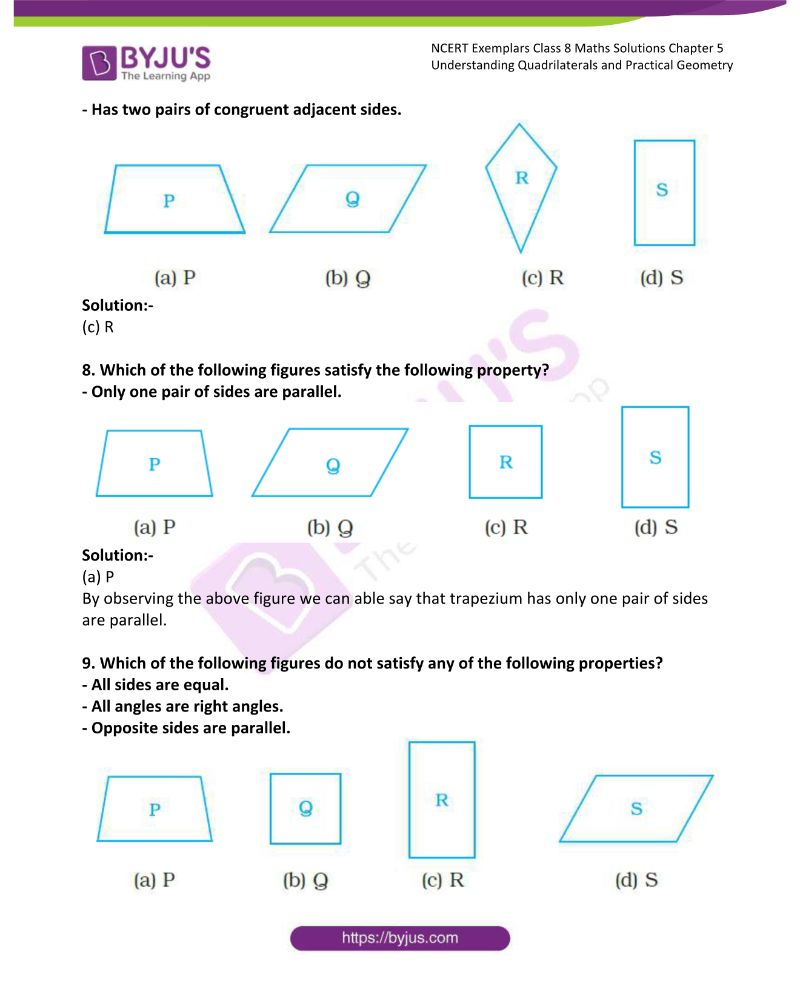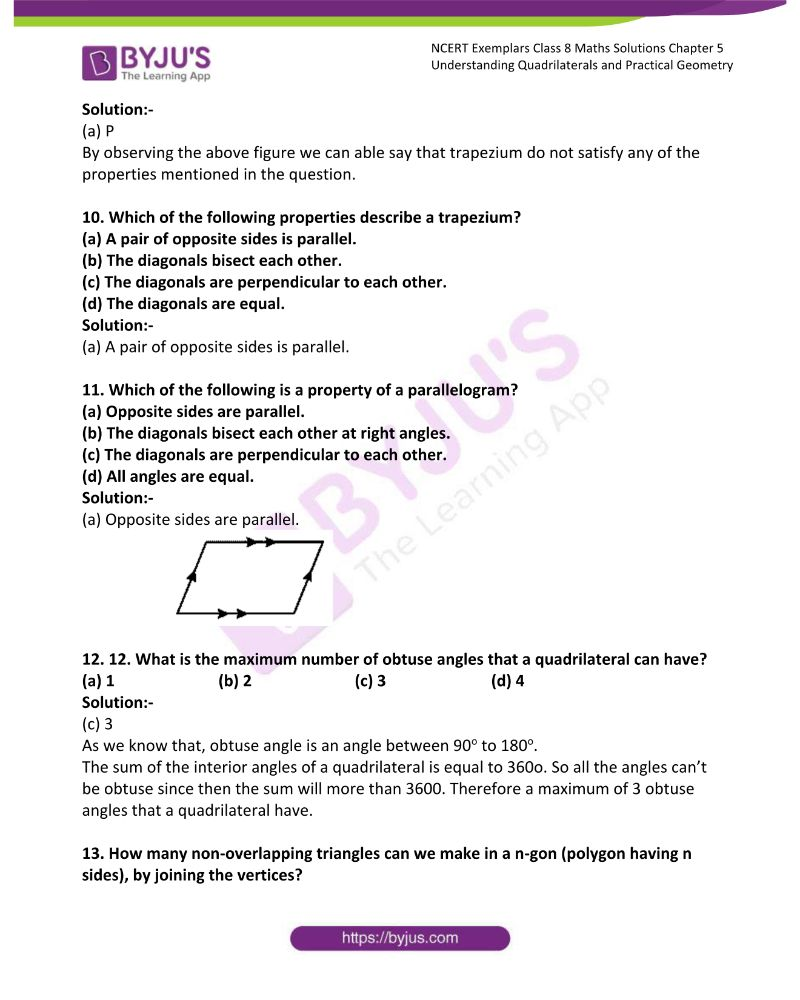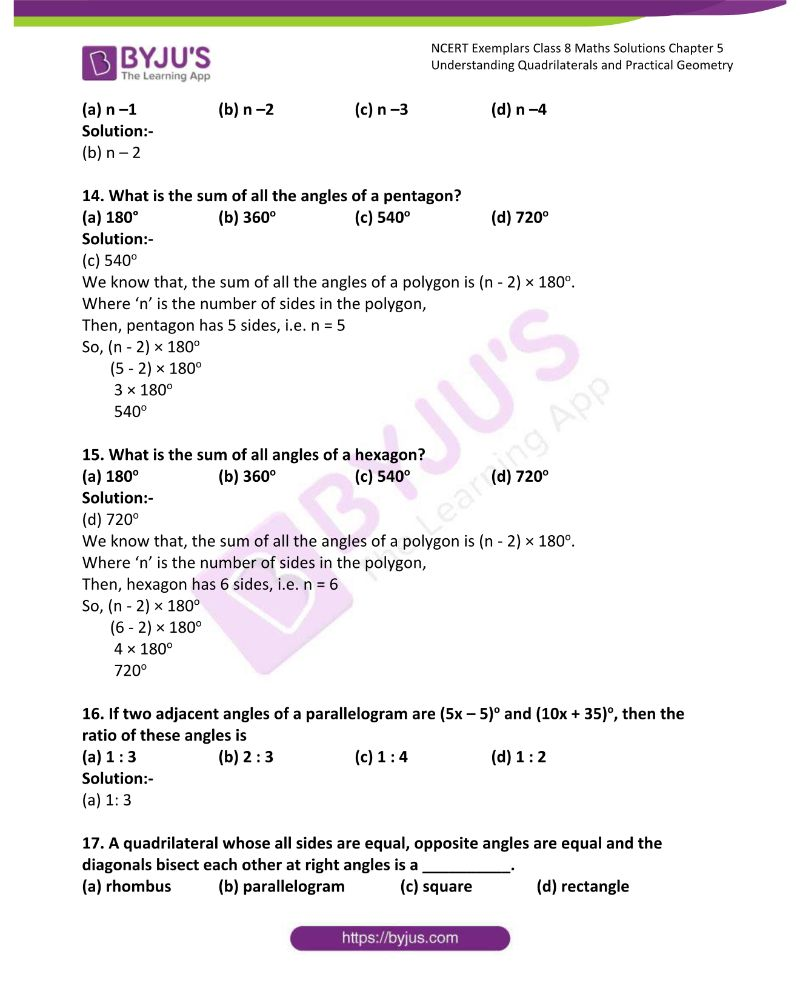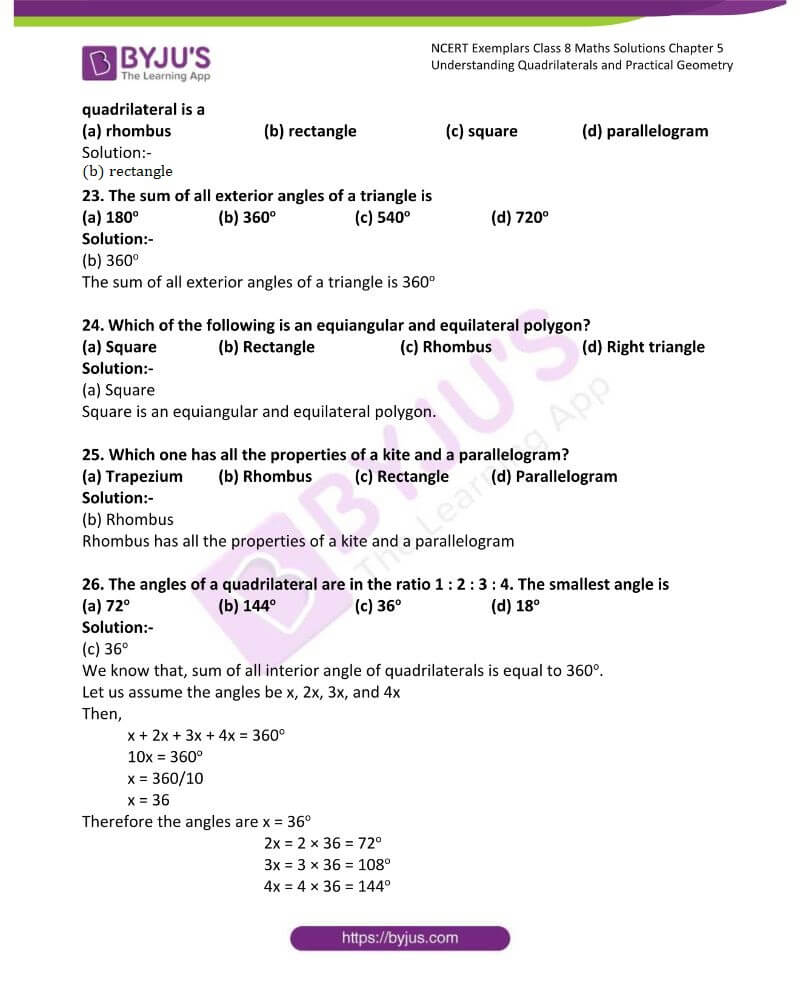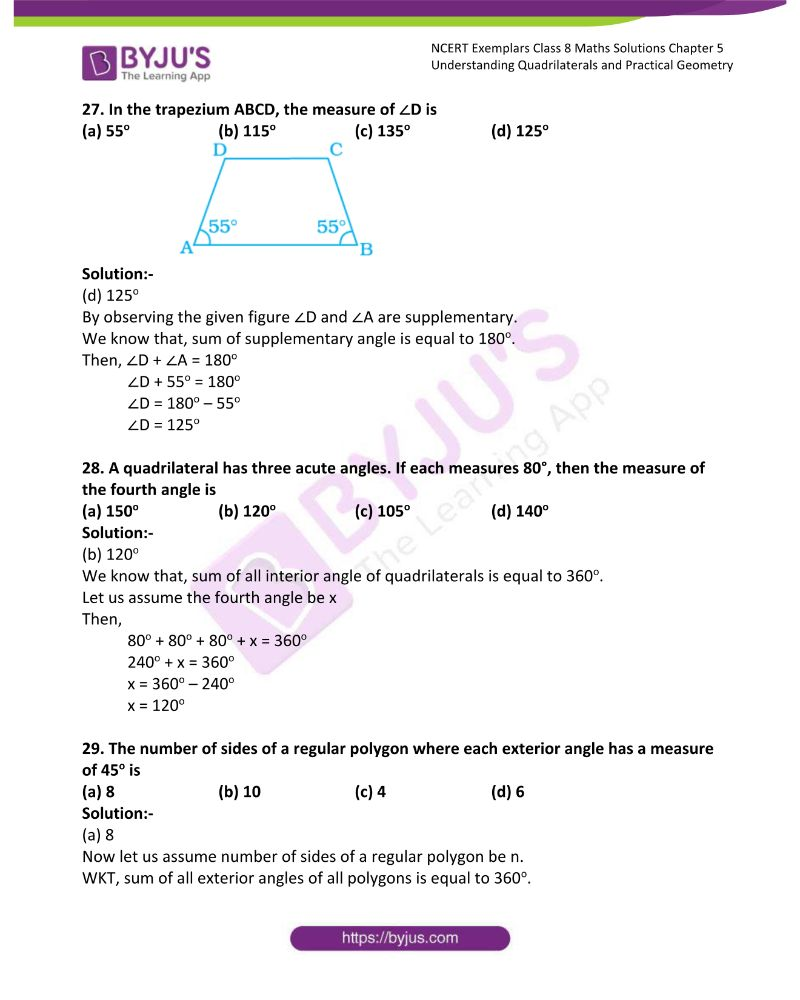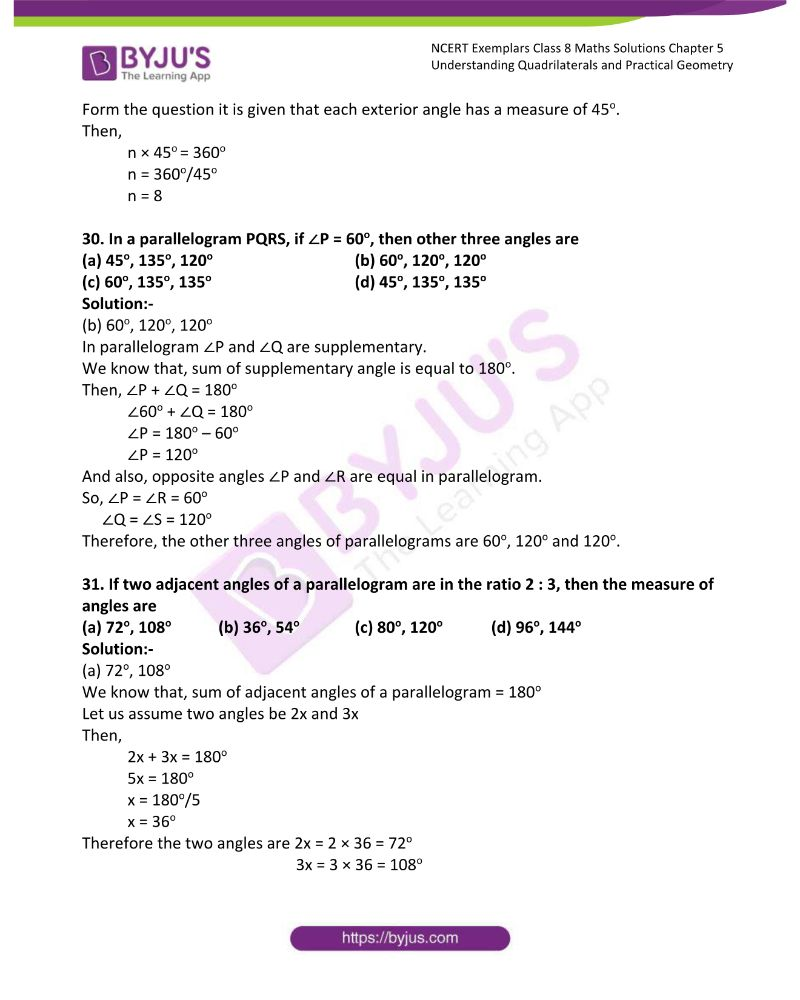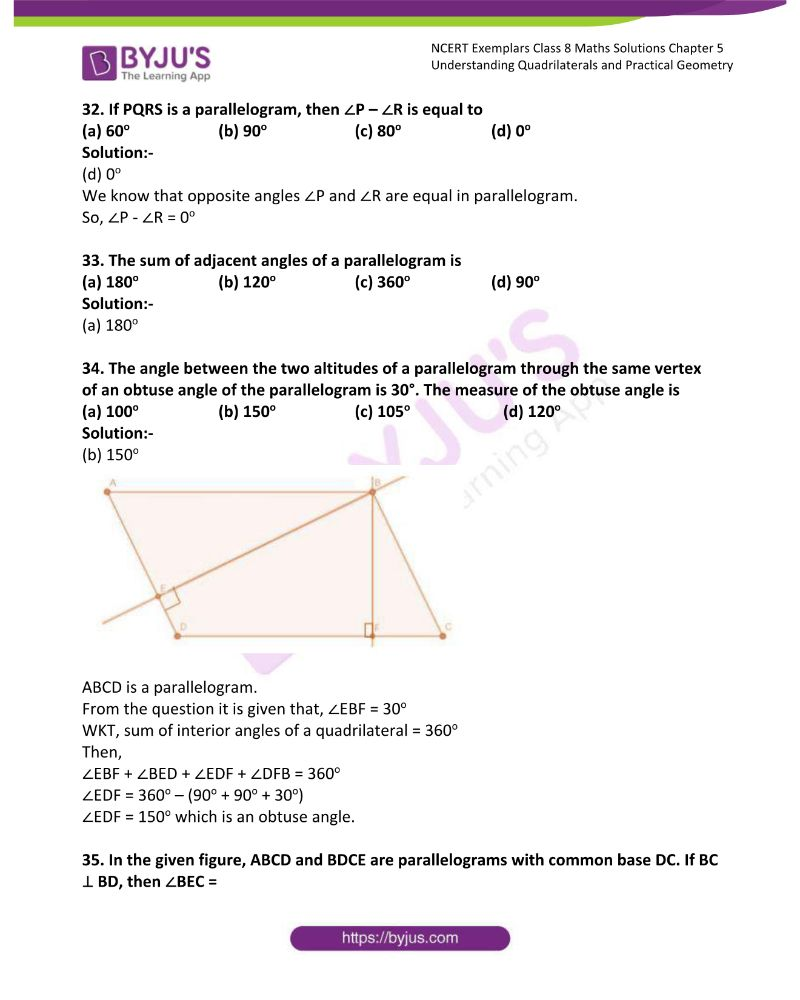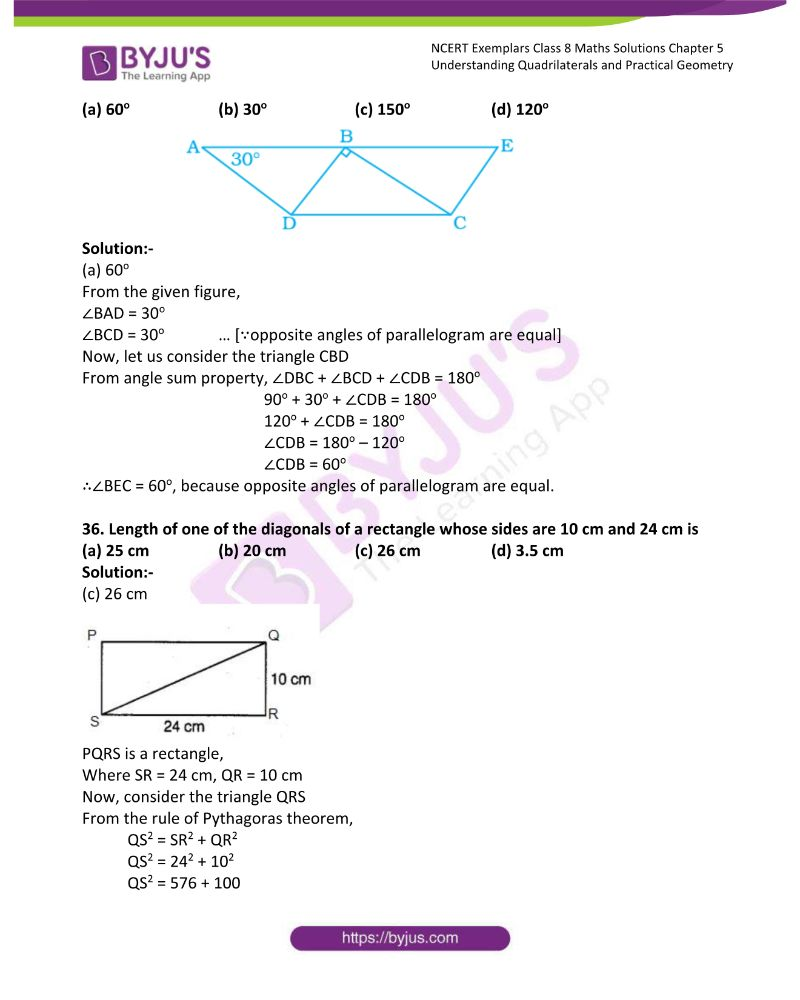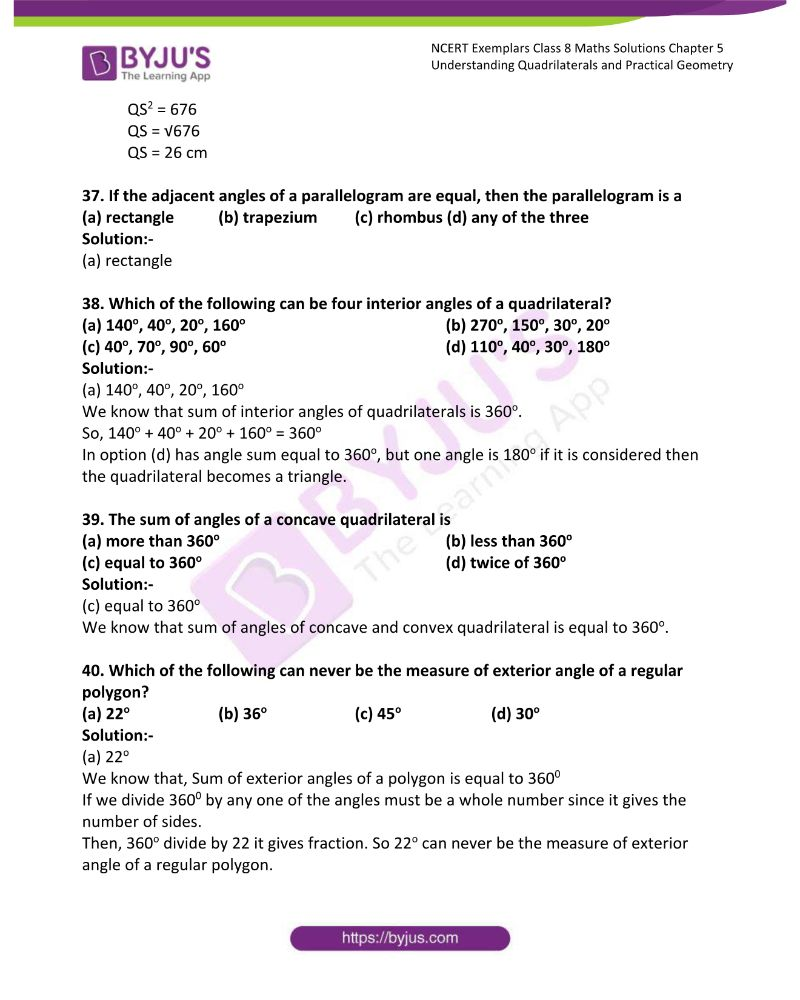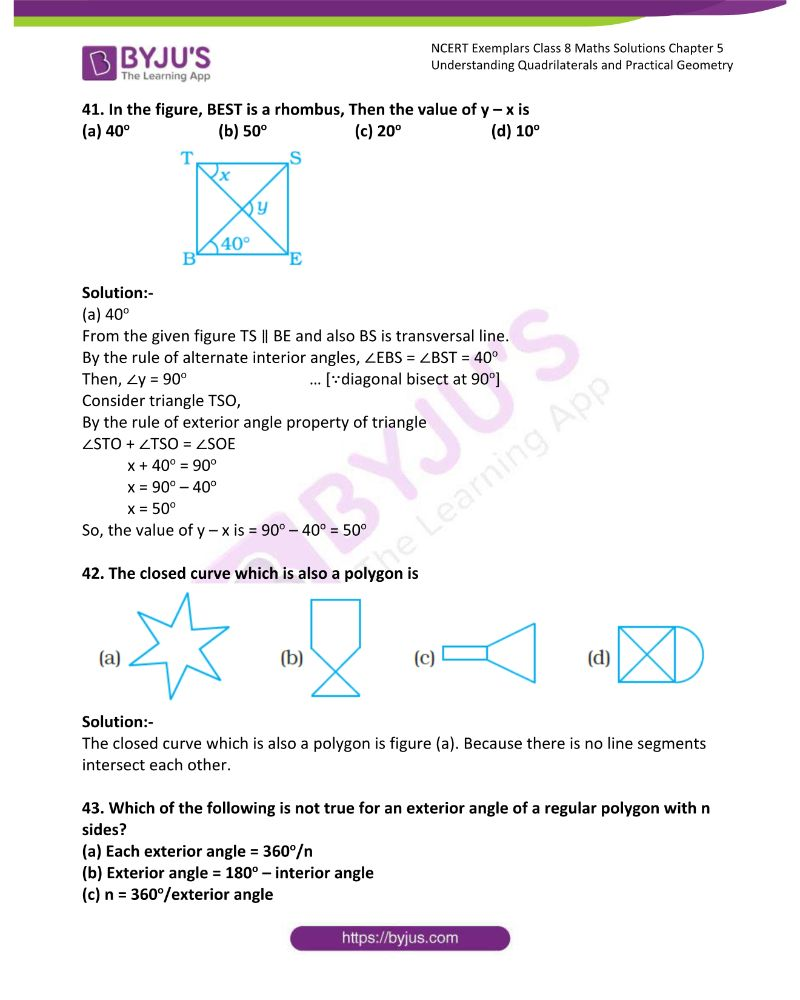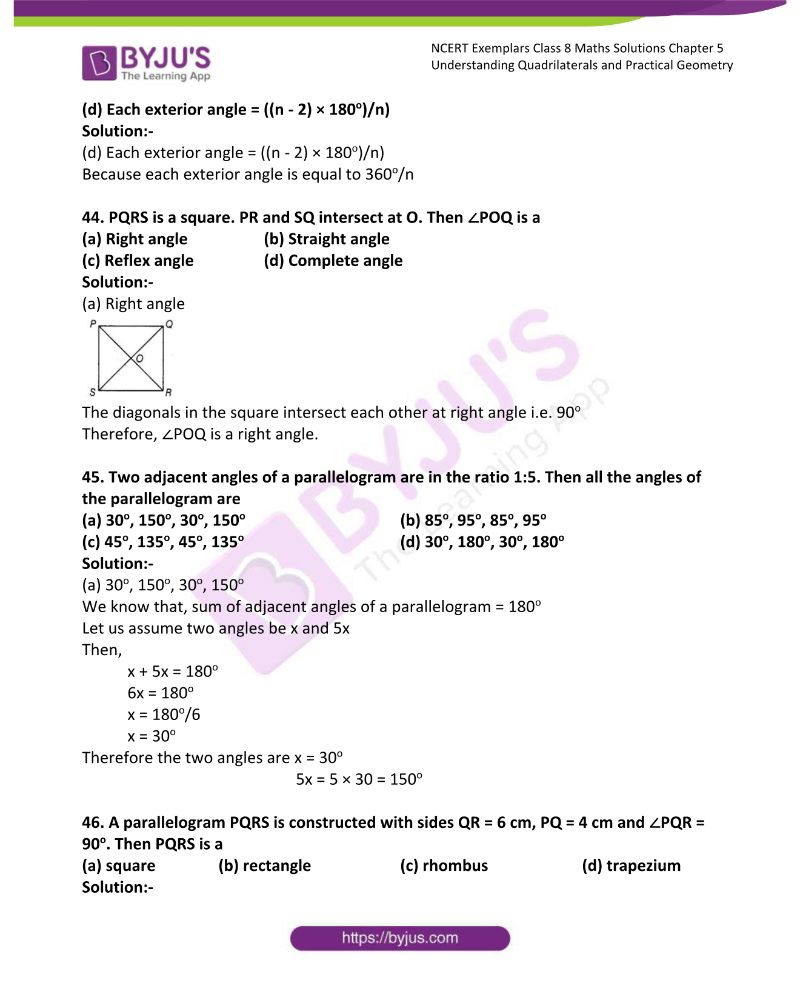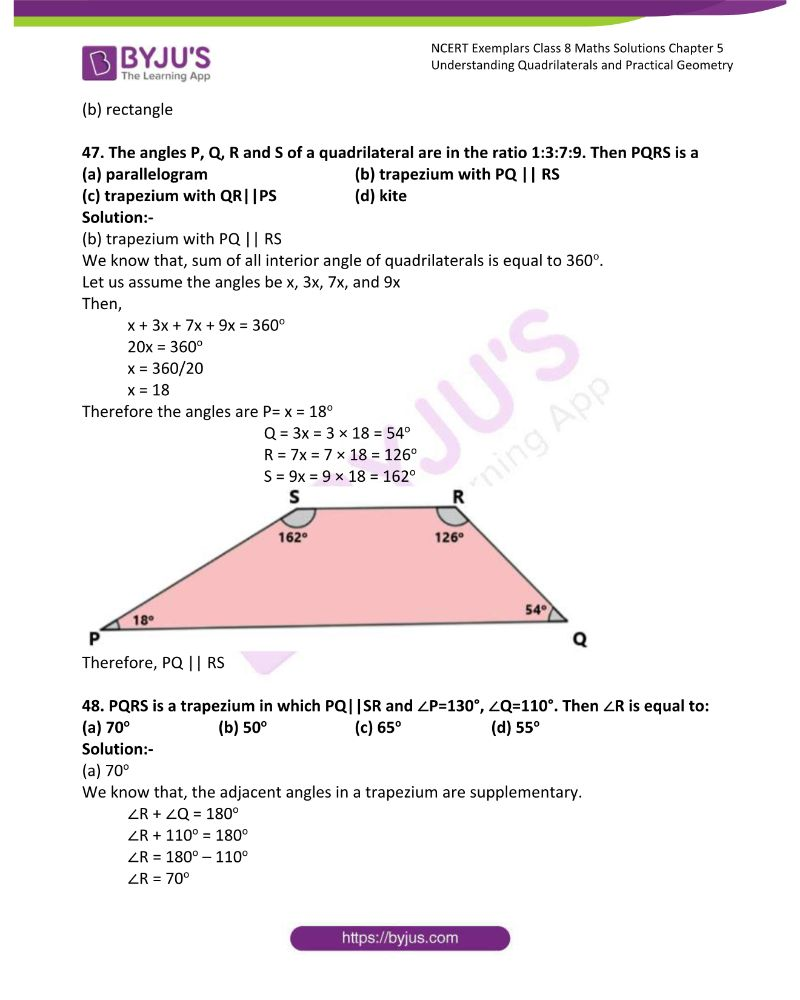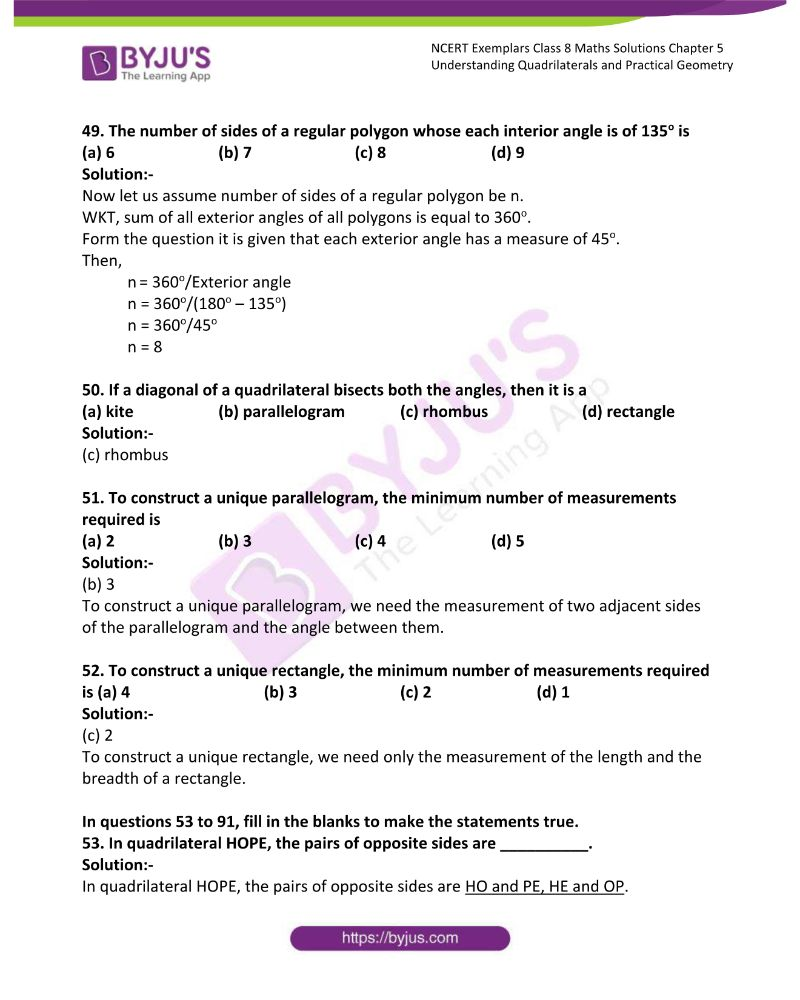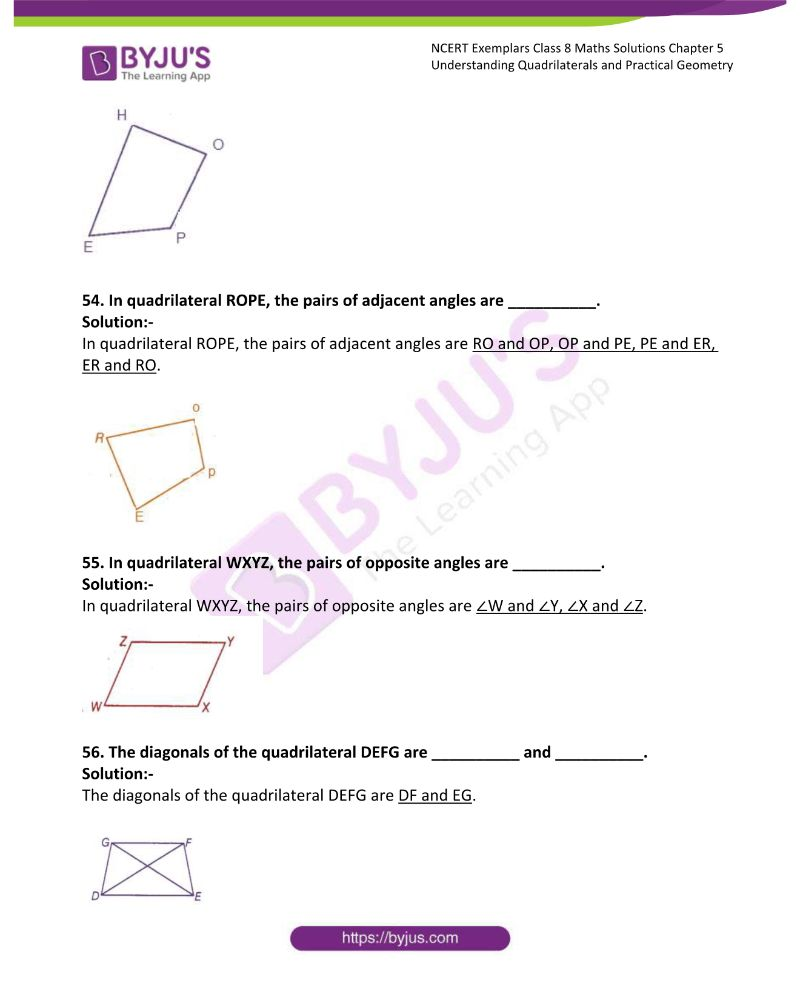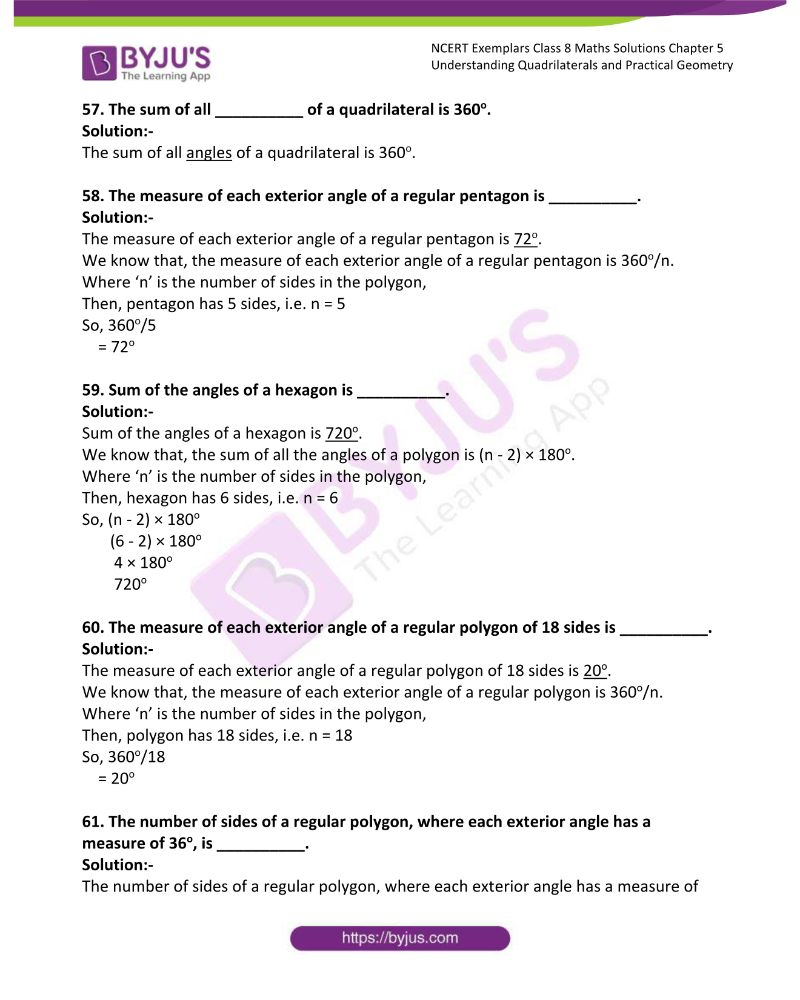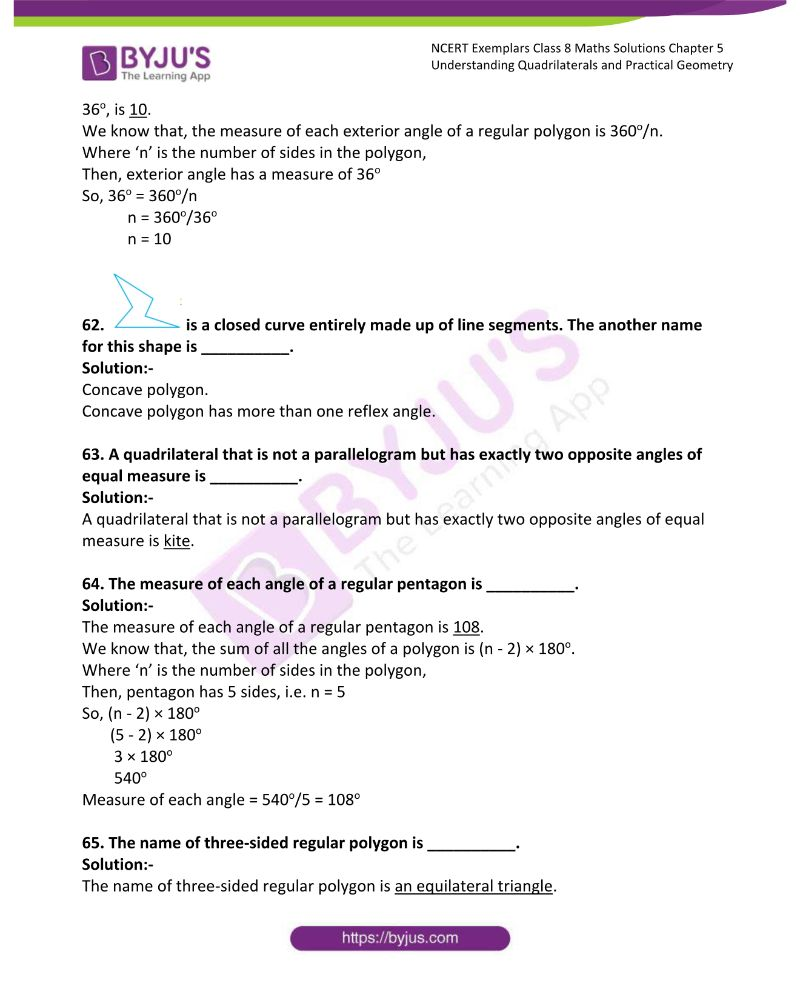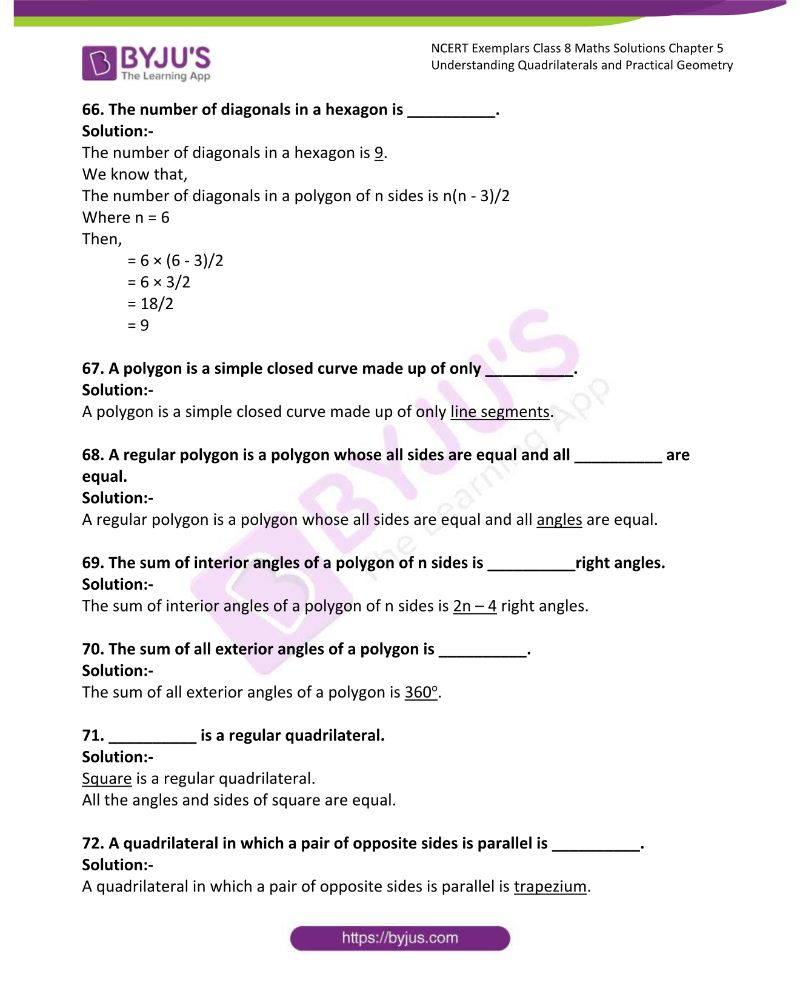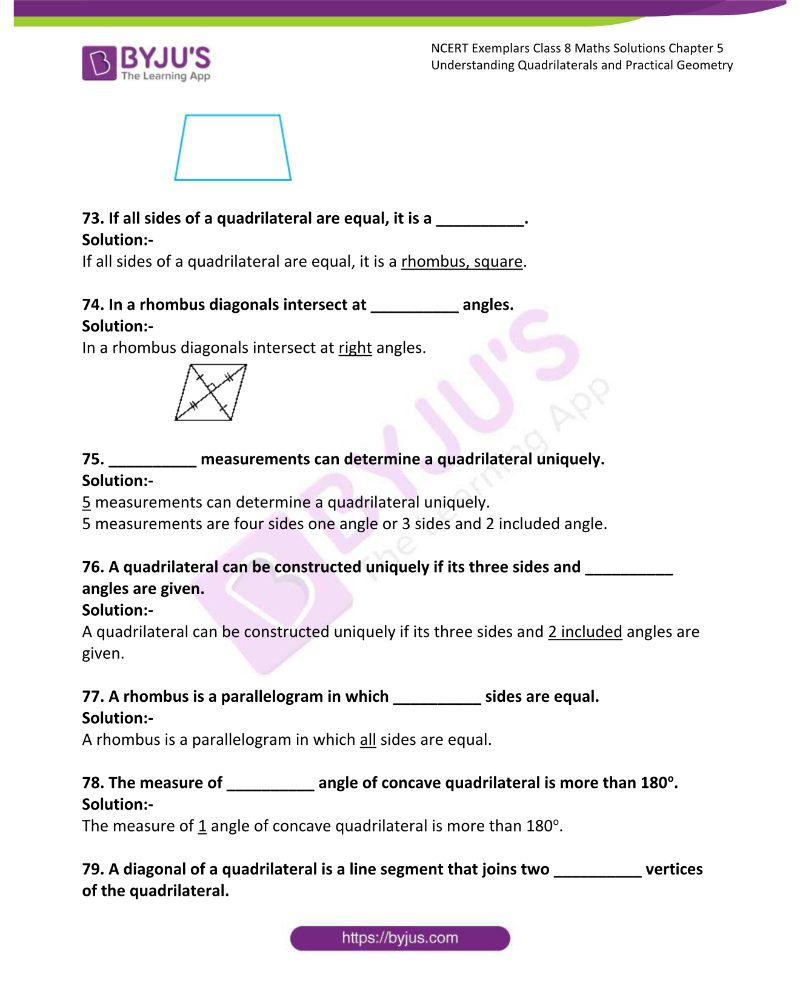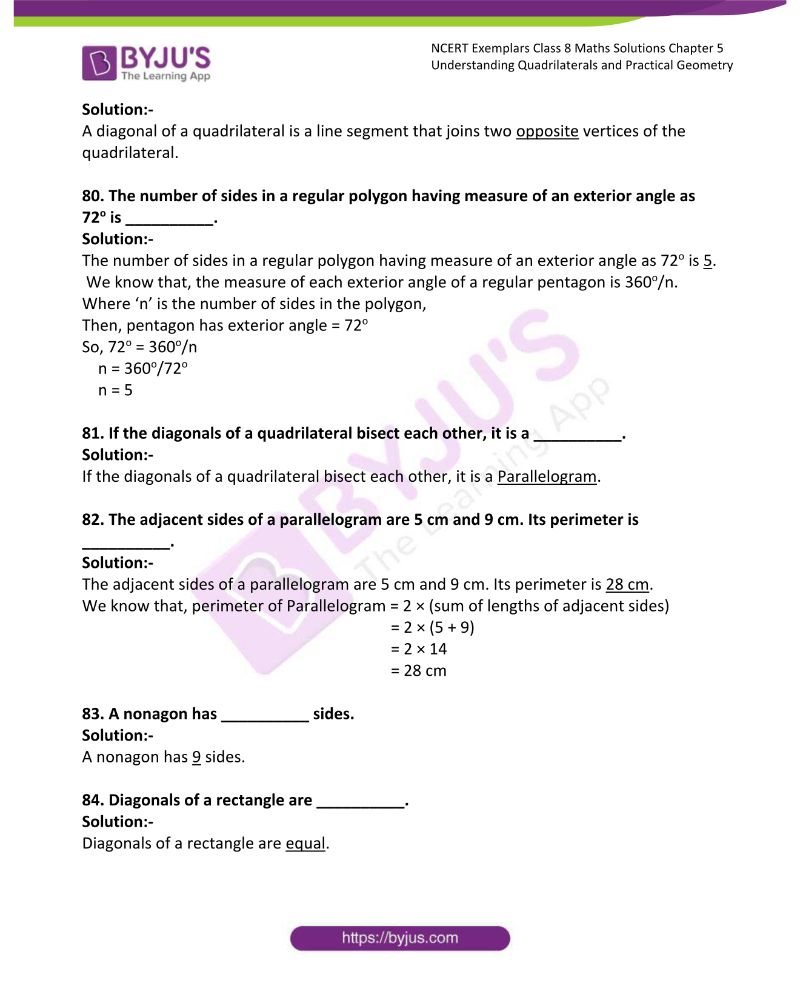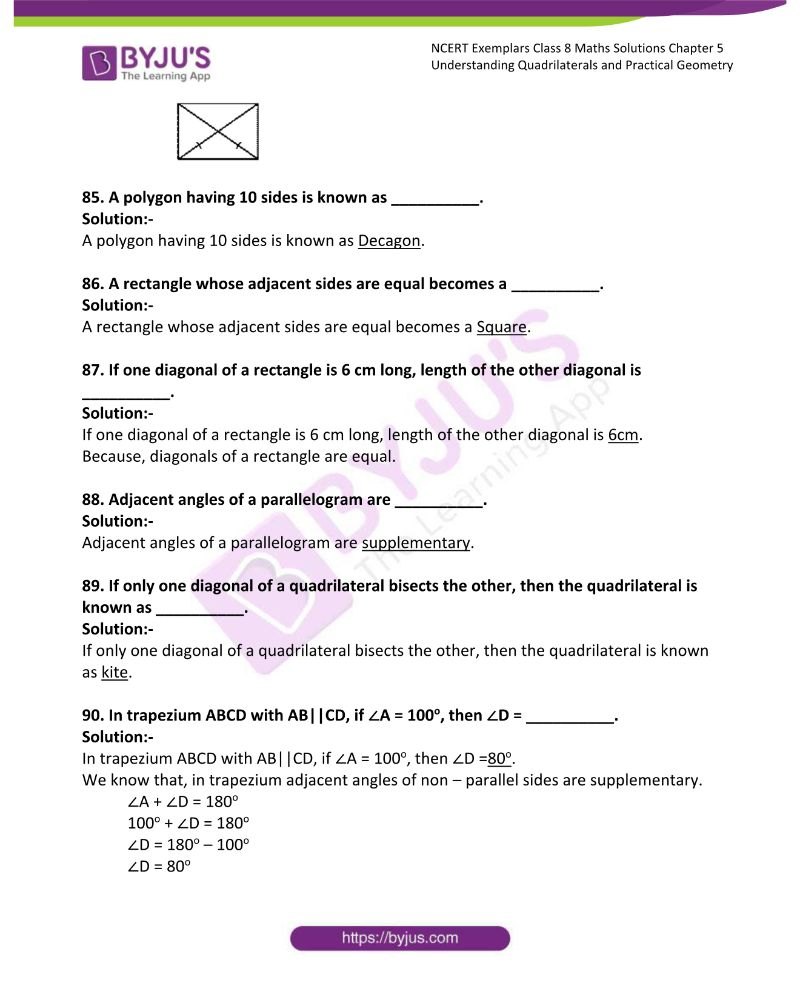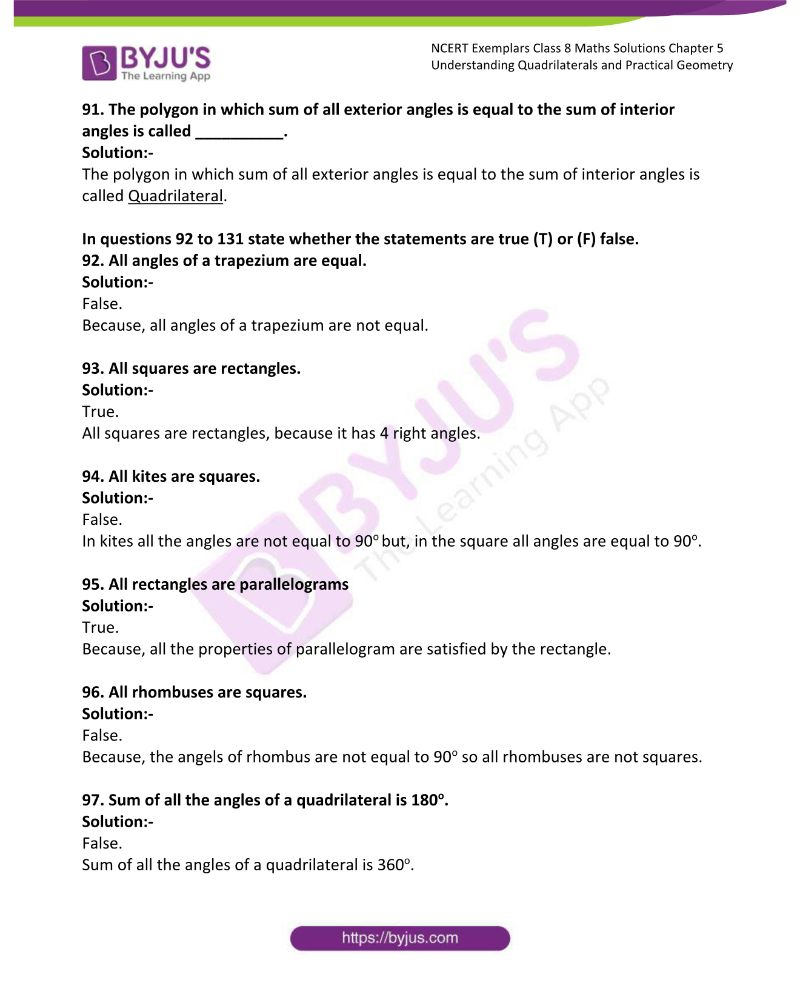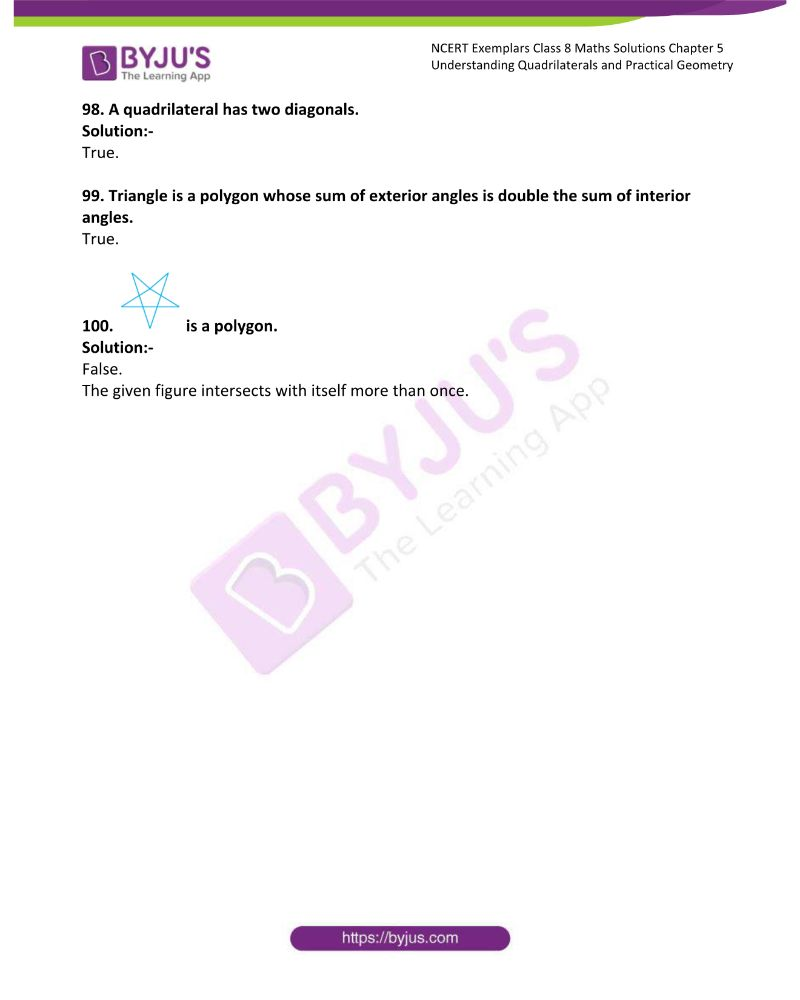### Access Answers to NCERT Exemplar Solutions Class 8 Maths Chapter 5

Exercise Page: 110

In questions 1 to 52, there are four options, out of which one is correct. Write the correct answer.

1. If three angles of a quadrilateral are each equal to 75o, the fourth angle is

(a) 150o (b) 135o (c) 45o (d) 75o

Solution:-

(b) 135o

We know that, sum of interior angles of quadrilateral is equal to 360o.

From the question it is given that, three angles of a quadrilateral are each equal to 75o.

Let us assume the fourth angle be x.

Then, 75o + 75o + 75o + x = 360o

225 + x = 360o

x = 360o – 225

x = 135o

2. For which of the following, diagonals bisect each other?

(a) Square (b) Kite (c) Trapezium (d) Quadrilateral

Solution:-

(a) Square3. For which of the following figures, all angles are equal?

(a) Rectangle (b) Kite (c) Trapezium (d) Rhombus

Solution:-

(a) Rectangle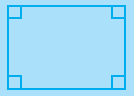So, in rectangle all angles are equal to 90o.

4. For which of the following figures, diagonals are perpendicular to each other?

(a) Parallelogram (b) Kite

(c) Trapezium (d) Rectangle

Solution:-

(b) Kite

In kite, diagonals are perpendicular to each other is as shown in the figure below.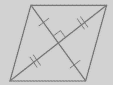5. For which of the following figures, diagonals are equal?

(a) Trapezium (b) Rhombus

(c) Parallelogram (d) Rectangle

Solution:-

(d) Rectangle

For rectangle, diagonals are equal is as shown in the figure below.6. Which of the following figures satisfy the following properties?

– All sides are congruent.

– All angles are right angles.

– Opposite sides are parallel.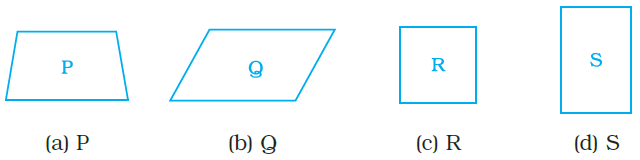Solution:-

(c) R

So, square has all sides are congruent, all angles are right angles and opposite sides are parallel.

7. Which of the following figures satisfy the following property?

– Has two pairs of congruent adjacent sides.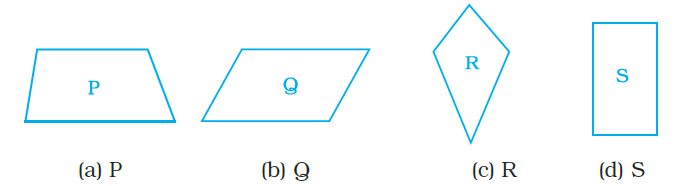Solution:-

(c) R

8. Which of the following figures satisfy the following property?

– Only one pair of sides are parallel.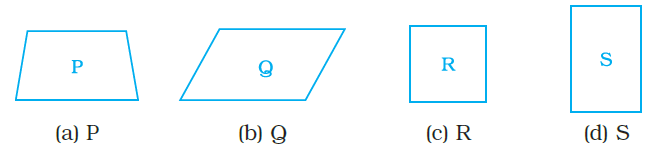Solution:-

(a) P

By observing the above figure we can able say that trapezium has only one pair of sides are parallel.

9. Which of the following figures do not satisfy any of the following properties?

– All sides are equal.

– All angles are right angles.

– Opposite sides are parallel.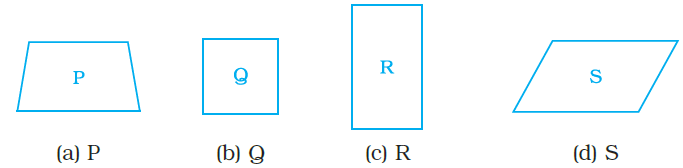Solution:-

(a) P

By observing the above figure we can able say that trapezium do not satisfy any of the properties mentioned in the question.

10. Which of the following properties describe a trapezium?

(a) A pair of opposite sides is parallel.

(b) The diagonals bisect each other.

(c) The diagonals are perpendicular to each other.

(d) The diagonals are equal.

Solution:-

(a) A pair of opposite sides is parallel.

11. Which of the following is a property of a parallelogram?

(a) Opposite sides are parallel.

(b) The diagonals bisect each other at right angles.

(c) The diagonals are perpendicular to each other.

(d) All angles are equal.

Solution:-

(a) Opposite sides are parallel.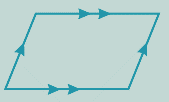12. 12. What is the maximum number of obtuse angles that a quadrilateral can have?

(a) 1 (b) 2 (c) 3 (d) 4

Solution:-

(c) 3

As we know that, obtuse angle is an angle between 90o to 180o.

The sum of the interior angles of a quadrilateral is equal to 360o. So all the angles can’t be obtuse since then the sum will more than 3600. Therefore a maximum of 3 obtuse angles that a quadrilateral have.

13. How many non-overlapping triangles can we make in a n-gon (polygon having n sides), by joining the vertices?

(a) n –1 (b) n –2 (c) n –3 (d) n –4

Solution:-

(b) n – 2

14. What is the sum of all the angles of a pentagon?

(a) 180° (b) 360o (c) 540o (d) 720o

Solution:-

(c) 540o

We know that, the sum of all the angles of a polygon is (n – 2) × 180o.

Where ‘n’ is the number of sides in the polygon,

Then, pentagon has 5 sides, i.e. n = 5

So, (n – 2) × 180o

(5 – 2) × 180o

3 × 180o

540o

15. What is the sum of all angles of a hexagon?

(a) 180o (b) 360o (c) 540o (d) 720o

Solution:-

(d) 720o

We know that, the sum of all the angles of a polygon is (n – 2) × 180o.

Where ‘n’ is the number of sides in the polygon,

Then, hexagon has 6 sides, i.e. n = 6

So, (n – 2) × 180o

(6 – 2) × 180o

4 × 180o

720o

16. If two adjacent angles of a parallelogram are (5x – 5)o and (10x + 35)o, then the ratio of these angles is

(a) 1 : 3 (b) 2 : 3 (c) 1 : 4 (d) 1 : 2

Solution:-

(a) 1: 3

17. A quadrilateral whose all sides are equal, opposite angles are equal and the diagonals bisect each other at right angles is a __________.

(a) rhombus (b) parallelogram (c) square (d) rectangle

Solution:-

(a) rhombus

A quadrilateral whose all sides are equal, opposite angles are equal and the diagonals bisect each other at right angles is a rhombus.

18. A quadrilateral whose opposite sides and all the angles are equal is a

(a) rectangle (b) parallelogram (c) square (d) rhombus

Solution:-

(a) rectangle

19. A quadrilateral whose all sides, diagonals and angles are equal is a

(a) square (b) trapezium (c) rectangle (d) rhombus

Solution:-

(a) Square

20. How many diagonals does a hexagon have?

(a) 9 (b) 8 (c) 2 (d) 6

Solution:-

(a) 9

We know that,

The number of diagonals in a polygon of n sides is n(n – 3)/2

Where n = 6

Then,

= 6 × (6 – 3)/2

= 6 × 3/2

= 18/2

= 9

21. If the adjacent sides of a parallelogram are equal then parallelogram is a

(a) rectangle (b) trapezium (c) rhombus (d) square

Solution:-

(c) rhombus22. If the diagonals of a quadrilateral are equal and bisect each other, then the quadrilateral is a

(a) rhombus (b) rectangle (c) square (d) parallelogram

Solution:-

(b) rectangle

23. The sum of all exterior angles of a triangle is

(a) 180o (b) 360o (c) 540o (d) 720o

Solution:-

(b) 360o

The sum of all exterior angles of a triangle is 360o

24. Which of the following is an equiangular and equilateral polygon?

(a) Square (b) Rectangle (c) Rhombus (d) Right triangle

Solution:-

(a) Square

Square is an equiangular and equilateral polygon.

25. Which one has all the properties of a kite and a parallelogram?

(a) Trapezium (b) Rhombus (c) Rectangle (d) Parallelogram

Solution:-

(b) Rhombus

Rhombus has all the properties of a kite and a parallelogram

26. The angles of a quadrilateral are in the ratio 1 : 2 : 3 : 4. The smallest angle is

(a) 72o (b) 144o (c) 36o (d) 18o

Solution:-

(c) 36o

We know that, sum of all interior angle of quadrilaterals is equal to 360o.

Let us assume the angles be x, 2x, 3x, and 4x

Then,

x + 2x + 3x + 4x = 360o

10x = 360o

x = 360/10

x = 36

Therefore the angles are x = 36o

2x = 2 × 36 = 72o

3x = 3 × 36 = 108o

4x = 4 × 36 = 144o

27. In the trapezium ABCD, the measure of ∠D is

(a) 55o (b) 115o (c) 135o (d) 125o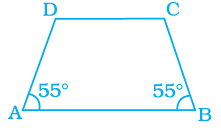Solution:-

(d) 125o

By observing the given figure ∠D and ∠A are supplementary.

We know that, sum of supplementary angle is equal to 180o.

Then, ∠D + ∠A = 180o

∠D + 55o = 180o

∠D = 180o – 55o

∠D = 125o

28. A quadrilateral has three acute angles. If each measures 80°, then the measure of the fourth angle is

(a) 150o (b) 120o (c) 105o (d) 140o

Solution:-

(b) 120o

We know that, sum of all interior angle of quadrilaterals is equal to 360o.

Let us assume the fourth angle be x

Then,

80o + 80o + 80o + x = 360o

240o + x = 360o

x = 360o – 240o

x = 120o

29. The number of sides of a regular polygon where each exterior angle has a measure of 45o is

(a) 8 (b) 10 (c) 4 (d) 6

Solution:-

(a) 8

Now let us assume number of sides of a regular polygon be n.

WKT, sum of all exterior angles of all polygons is equal to 360o.

Form the question it is given that each exterior angle has a measure of 45o.

Then,

n × 45o = 360o

n = 360o/45o

n = 8

30. In a parallelogram PQRS, if ∠P = 60o, then other three angles are

(a) 45o, 135o, 120o (b) 60o, 120o, 120o

(c) 60o, 135o, 135o (d) 45o, 135o, 135o

Solution:-

(b) 60o, 120o, 120o

In parallelogram ∠P and ∠Q are supplementary.

We know that, sum of supplementary angle is equal to 180o.

Then, ∠P + ∠Q = 180o

∠60o + ∠Q = 180o

∠P = 180o – 60o

∠P = 120o

And also, opposite angles ∠P and ∠R are equal in parallelogram.

So, ∠P = ∠R = 60o

∠Q = ∠S = 120o

Therefore, the other three angles of parallelograms are 60o, 120o and 120o.

31. If two adjacent angles of a parallelogram are in the ratio 2 : 3, then the measure of angles are

(a) 72o, 108o (b) 36o, 54o (c) 80o, 120o (d) 96o, 144o

Solution:-

(a) 72o, 108o

We know that, sum of adjacent angles of a parallelogram = 180o

Let us assume two angles be 2x and 3x

Then,

2x + 3x = 180o

5x = 180o

x = 180o/5

x = 36o

Therefore the two angles are 2x = 2 × 36 = 72o

3x = 3 × 36 = 108o

32. If PQRS is a parallelogram, then ∠P – ∠R is equal to

(a) 60o (b) 90o (c) 80o (d) 0o

Solution:-

(d) 0o

We know that opposite angles ∠P and ∠R are equal in parallelogram.

So, ∠P – ∠R = 0o

33. The sum of adjacent angles of a parallelogram is

(a) 180o (b) 120o (c) 360o (d) 90o

Solution:-

(a) 180o

34. The angle between the two altitudes of a parallelogram through the same vertex of an obtuse angle of the parallelogram is 30°. The measure of the obtuse angle is

(a) 100o (b) 150o (c) 105o (d) 120o

Solution:-

(b) 150o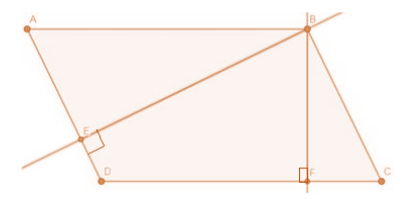ABCD is a parallelogram.

From the question it is given that, ∠EBF = 30o

WKT, sum of interior angles of a quadrilateral = 360o

Then,

∠EBF + ∠BED + ∠EDF + ∠DFB = 360o

∠EDF = 360o – (90o + 90o + 30o)

∠EDF = 150o which is an obtuse angle.

35. In the given figure, ABCD and BDCE are parallelograms with common base DC. If BC ⊥ BD, then ∠BEC =

(a) 60o (b) 30o (c) 150o (d) 120o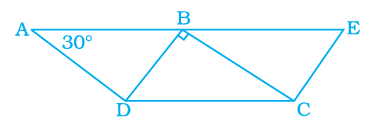Solution:-

(a) 60o

From the given figure,

∠BCD = 30o … [∵opposite angles of parallelogram are equal]

Now, let us consider the triangle CBD

From angle sum property, ∠DBC + ∠BCD + ∠CDB = 180o

90o + 30o + ∠CDB = 180o

120o + ∠CDB = 180o

∠CDB = 180o – 120o

∠CDB = 60o

∴∠BEC = 60o, because opposite angles of parallelogram are equal.

36. Length of one of the diagonals of a rectangle whose sides are 10 cm and 24 cm is

(a) 25 cm (b) 20 cm (c) 26 cm (d) 3.5 cm

Solution:-

(c) 26 cm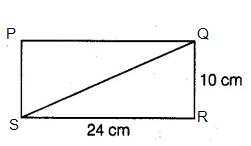PQRS is a rectangle,

Where SR = 24 cm, QR = 10 cm

Now, consider the triangle QRS

From the rule of Pythagoras theorem,

QS2 = SR2 + QR2

QS2 = 242 + 102

QS2 = 576 + 100

QS2 = 676

QS = √676

QS = 26 cm

37. If the adjacent angles of a parallelogram are equal, then the parallelogram is a

(a) rectangle (b) trapezium (c) rhombus (d) any of the three

Solution:-

(a) rectangle

38. Which of the following can be four interior angles of a quadrilateral?

(a) 140o, 40o, 20o, 160o (b) 270o, 150o, 30o, 20o

(c) 40o, 70o, 90o, 60o (d) 110o, 40o, 30o, 180o

Solution:-

(a) 140o, 40o, 20o, 160o

We know that sum of interior angles of quadrilaterals is 360o.

So, 140o + 40o + 20o + 160o = 360o

In option (d) has angle sum equal to 360o, but one angle is 180o if it is considered then the quadrilateral becomes a triangle.

39. The sum of angles of a concave quadrilateral is

(a) more than 360o (b) less than 360o

(c) equal to 360o (d) twice of 360o

Solution:-

(c) equal to 360o

We know that sum of angles of concave and convex quadrilateral is equal to 360o.

40. Which of the following can never be the measure of exterior angle of a regular polygon?

(a) 22o (b) 36o (c) 45o (d) 30o

Solution:-

(a) 22o

We know that, Sum of exterior angles of a polygon is equal to 3600

If we divide 3600 by any one of the angles must be a whole number since it gives the number of sides.

Then, 360o divide by 22 it gives fraction. So 22o can never be the measure of exterior angle of a regular polygon.

41. In the figure, BEST is a rhombus, Then the value of y – x is

(a) 40o (b) 50o (c) 20o (d) 10o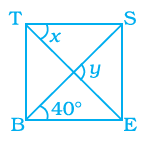Solution:-

(a) 40o

From the given figure TS ∥ BE and also BS is transversal line.

By the rule of alternate interior angles, ∠EBS = ∠BST = 40o

Then, ∠y = 90o … [∵diagonal bisect at 90o]

Consider triangle TSO,

By the rule of exterior angle property of triangle

∠STO + ∠TSO = ∠SOE

x + 40o = 90o

x = 90o – 40o

x = 50o

So, the value of y – x is = 90o – 40o = 50o

42. The closed curve which is also a polygon is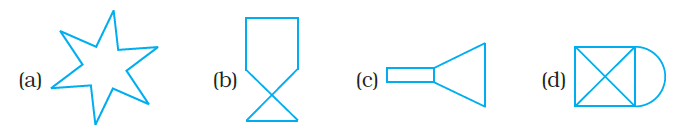Solution:-

The closed curve which is also a polygon is figure (a). Because there is no line segments intersect each other.

43. Which of the following is not true for an exterior angle of a regular polygon with n sides?

(a) Each exterior angle = 360o/n

(b) Exterior angle = 180o – interior angle

(c) n = 360o/exterior angle

(d) Each exterior angle = ((n – 2) × 180o)/n)

Solution:-

(d) Each exterior angle = ((n – 2) × 180o)/n)

Because each exterior angle is equal to 360o/n

44. PQRS is a square. PR and SQ intersect at O. Then ∠POQ is a

(a) Right angle (b) Straight angle

(c) Reflex angle (d) Complete angle

Solution:-

(a) Right angle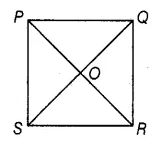The diagonals in the square intersect each other at right angle i.e. 90o

Therefore, ∠POQ is a right angle.

45. Two adjacent angles of a parallelogram are in the ratio 1:5. Then all the angles of the parallelogram are

(a) 30o, 150o, 30o, 150o (b) 85o, 95o, 85o, 95o

(c) 45o, 135o, 45o, 135o (d) 30o, 180o, 30o, 180o

Solution:-

(a) 30o, 150o, 30o, 150o

We know that, sum of adjacent angles of a parallelogram = 180o

Let us assume two angles be x and 5x

Then,

x + 5x = 180o

6x = 180o

x = 180o/6

x = 30o

Therefore the two angles are x = 30o

5x = 5 × 30 = 150o

46. A parallelogram PQRS is constructed with sides QR = 6 cm, PQ = 4 cm and ∠PQR = 90o. Then PQRS is a

(a) square (b) rectangle (c) rhombus (d) trapezium

Solution:-

(b) rectangle

47. The angles P, Q, R and S of a quadrilateral are in the ratio 1:3:7:9. Then PQRS is a

(a) parallelogram (b) trapezium with PQ || RS

(c) trapezium with QR||PS (d) kite

Solution:-

(b) trapezium with PQ || RS

We know that, sum of all interior angle of quadrilaterals is equal to 360o.

Let us assume the angles be x, 3x, 7x, and 9x

Then,

x + 3x + 7x + 9x = 360o

20x = 360o

x = 360/20

x = 18

Therefore the angles are P= x = 18o

Q = 3x = 3 × 18 = 54o

R = 7x = 7 × 18 = 126o

S = 9x = 9 × 18 = 162o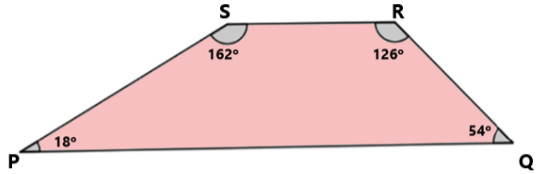Therefore, PQ || RS

48. PQRS is a trapezium in which PQ||SR and ∠P=130°, ∠Q=110°. Then ∠R is equal to: (a) 70o (b) 50o (c) 65o (d) 55o

Solution:-

(a) 70o

We know that, the adjacent angles in a trapezium are supplementary.

∠R + ∠Q = 180o

∠R + 110o = 180o

∠R = 180o – 110o

∠R = 70o

49. The number of sides of a regular polygon whose each interior angle is of 135o is

(a) 6 (b) 7 (c) 8 (d) 9

Solution:-

Now let us assume number of sides of a regular polygon be n.

WKT, sum of all exterior angles of all polygons is equal to 360o.

Form the question it is given that each exterior angle has a measure of 45o.

Then,

n = 360o/Exterior angle

n = 360o/(180o – 135o)

n = 360o/45o

n = 8

50. If a diagonal of a quadrilateral bisects both the angles, then it is a

(a) kite (b) parallelogram (c) rhombus (d) rectangle

Solution:-

(c) rhombus

51. To construct a unique parallelogram, the minimum number of measurements required is

(a) 2 (b) 3 (c) 4 (d) 5

Solution:-

(b) 3

To construct a unique parallelogram, we need the measurement of two adjacent sides of the parallelogram and the angle between them.

52. To construct a unique rectangle, the minimum number of measurements required is

(a) 4 (b) 3 (c) 2 (d) 1

Solution:-

(c) 2

To construct a unique rectangle, we need only the measurement of the length and the breadth of a rectangle.

In questions 53 to 91, fill in the blanks to make the statements true.

53. In quadrilateral HOPE, the pairs of opposite sides are __________.

Solution:-

In quadrilateral HOPE, the pairs of opposite sides are HO and PE, HE and OP.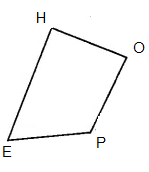Solution:-

In quadrilateral ROPE, the pairs of adjacent angles are RO and OP, OP and PE, PE and ER, ER and RO.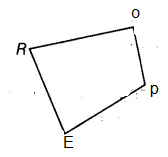55. In quadrilateral WXYZ, the pairs of opposite angles are __________.

Solution:-

In quadrilateral WXYZ, the pairs of opposite angles are ∠W and ∠Y, ∠X and ∠Z.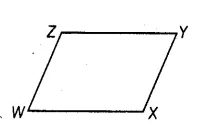56. The diagonals of the quadrilateral DEFG are __________ and __________.

Solution:-

The diagonals of the quadrilateral DEFG are DF and EG.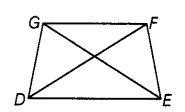57. The sum of all __________ of a quadrilateral is 360o.

Solution:-

The sum of all angles of a quadrilateral is 360o.

58. The measure of each exterior angle of a regular pentagon is __________.

Solution:-

The measure of each exterior angle of a regular pentagon is 72o.

We know that, the measure of each exterior angle of a regular pentagon is 360o/n.

Where ‘n’ is the number of sides in the polygon,

Then, pentagon has 5 sides, i.e. n = 5

So, 360o/5

= 72o

59. Sum of the angles of a hexagon is __________.

Solution:-

Sum of the angles of a hexagon is 720o.

We know that, the sum of all the angles of a polygon is (n – 2) × 180o.

Where ‘n’ is the number of sides in the polygon,

Then, hexagon has 6 sides, i.e. n = 6

So, (n – 2) × 180o

(6 – 2) × 180o

4 × 180o

720o

60. The measure of each exterior angle of a regular polygon of 18 sides is __________.

Solution:-

The measure of each exterior angle of a regular polygon of 18 sides is 20o.

We know that, the measure of each exterior angle of a regular polygon is 360o/n.

Where ‘n’ is the number of sides in the polygon,

Then, polygon has 18 sides, i.e. n = 18

So, 360o/18

= 20o

61. The number of sides of a regular polygon, where each exterior angle has a measure of 36o, is __________.

Solution:-

The number of sides of a regular polygon, where each exterior angle has a measure of 36o, is 10.

We know that, the measure of each exterior angle of a regular polygon is 360o/n.

Where ‘n’ is the number of sides in the polygon,

Then, exterior angle has a measure of 36o

So, 36o = 360o/n

n = 360o/36o

n = 10

62.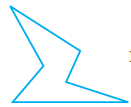is a closed curve entirely made up of line segments. The another name for this shape is __________.

Solution:-

Concave polygon.

Concave polygon has more than one reflex angle.

63. A quadrilateral that is not a parallelogram but has exactly two opposite angles of equal measure is __________.

Solution:-

A quadrilateral that is not a parallelogram but has exactly two opposite angles of equal measure is kite.

64. The measure of each angle of a regular pentagon is __________.

Solution:-

The measure of each angle of a regular pentagon is 108.

We know that, the sum of all the angles of a polygon is (n – 2) × 180o.

Where ‘n’ is the number of sides in the polygon,

Then, pentagon has 5 sides, i.e. n = 5

So, (n – 2) × 180o

(5 – 2) × 180o

3 × 180o

540o

Measure of each angle = 540o/5 = 108o

65. The name of three-sided regular polygon is __________.

Solution:-

The name of three-sided regular polygon is an equilateral triangle.

66. The number of diagonals in a hexagon is __________.

Solution:-

The number of diagonals in a hexagon is 9.

We know that,

The number of diagonals in a polygon of n sides is n(n – 3)/2

Where n = 6

Then,

= 6 × (6 – 3)/2

= 6 × 3/2

= 18/2

= 9

67. A polygon is a simple closed curve made up of only __________.

Solution:-

A polygon is a simple closed curve made up of only line segments.

68. A regular polygon is a polygon whose all sides are equal and all __________ are equal.

Solution:-

A regular polygon is a polygon whose all sides are equal and all angles are equal.

69. The sum of interior angles of a polygon of n sides is __________right angles.

Solution:-

The sum of interior angles of a polygon of n sides is 2n – 4 right angles.

70. The sum of all exterior angles of a polygon is __________.

Solution:-

The sum of all exterior angles of a polygon is 360o.

71. __________ is a regular quadrilateral.

Solution:-

All the angles and sides of square are equal.

72. A quadrilateral in which a pair of opposite sides is parallel is __________.

Solution:-

A quadrilateral in which a pair of opposite sides is parallel is trapezium.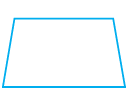73. If all sides of a quadrilateral are equal, it is a __________.

Solution:-

If all sides of a quadrilateral are equal, it is a rhombus, square.

74. In a rhombus diagonals intersect at __________ angles.

Solution:-

In a rhombus diagonals intersect at right angles.75. __________ measurements can determine a quadrilateral uniquely.

Solution:-

5 measurements can determine a quadrilateral uniquely.

5 measurements are four sides one angle or 3 sides and 2 included angle.

76. A quadrilateral can be constructed uniquely if its three sides and __________ angles are given.

Solution:-

A quadrilateral can be constructed uniquely if its three sides and 2 included angles are given.

77. A rhombus is a parallelogram in which __________ sides are equal.

Solution:-

A rhombus is a parallelogram in which all sides are equal.

78. The measure of __________ angle of concave quadrilateral is more than 180o.

Solution:-

The measure of 1 angle of concave quadrilateral is more than 180o.

79. A diagonal of a quadrilateral is a line segment that joins two __________ vertices of the quadrilateral.

Solution:-

A diagonal of a quadrilateral is a line segment that joins two opposite vertices of the quadrilateral.

80. The number of sides in a regular polygon having measure of an exterior angle as 72o is __________.

Solution:-

The number of sides in a regular polygon having measure of an exterior angle as 72o is 5.

We know that, the measure of each exterior angle of a regular pentagon is 360o/n.

Where ‘n’ is the number of sides in the polygon,

Then, pentagon has exterior angle = 72o

So, 72o = 360o/n

n = 360o/72o

n = 5

81. If the diagonals of a quadrilateral bisect each other, it is a __________.

Solution:-

If the diagonals of a quadrilateral bisect each other, it is a Parallelogram.

82. The adjacent sides of a parallelogram are 5 cm and 9 cm. Its perimeter is __________.

Solution:-

The adjacent sides of a parallelogram are 5 cm and 9 cm. Its perimeter is 28 cm.

We know that, perimeter of Parallelogram = 2 × (sum of lengths of adjacent sides)

= 2 × (5 + 9)

= 2 × 14

= 28 cm

83. A nonagon has __________ sides.

Solution:-

A nonagon has 9 sides.

84. Diagonals of a rectangle are __________.

Solution:-

Diagonals of a rectangle are equal.85. A polygon having 10 sides is known as __________.

Solution:-

A polygon having 10 sides is known as Decagon.

86. A rectangle whose adjacent sides are equal becomes a __________.

Solution:-

A rectangle whose adjacent sides are equal becomes a Square.

87. If one diagonal of a rectangle is 6 cm long, length of the other diagonal is __________.

Solution:-

If one diagonal of a rectangle is 6 cm long, length of the other diagonal is 6cm.

Because, diagonals of a rectangle are equal.

88. Adjacent angles of a parallelogram are __________.

Solution:-

Adjacent angles of a parallelogram are supplementary.

89. If only one diagonal of a quadrilateral bisects the other, then the quadrilateral is known as __________.

Solution:-

If only one diagonal of a quadrilateral bisects the other, then the quadrilateral is known as kite.

90. In trapezium ABCD with AB||CD, if ∠A = 100o, then ∠D = __________.

Solution:-

In trapezium ABCD with AB||CD, if ∠A = 100o, then ∠D =80o.

We know that, in trapezium adjacent angles of non – parallel sides are supplementary.

∠A + ∠D = 180o

100o + ∠D = 180o

∠D = 180o – 100o

∠D = 80o

91. The polygon in which sum of all exterior angles is equal to the sum of interior angles is called __________.

Solution:-

The polygon in which sum of all exterior angles is equal to the sum of interior angles is called Quadrilateral.

In questions 92 to 131 state whether the statements are true (T) or (F) false.

92. All angles of a trapezium are equal.

Solution:-

False.

Because, all angles of a trapezium are not equal.

93. All squares are rectangles.

Solution:-

True.

All squares are rectangles, because it has 4 right angles.

94. All kites are squares.

Solution:-

False.

In kites all the angles are not equal to 90o but, in the square all angles are equal to 90o.

95. All rectangles are parallelograms

Solution:-

True.

Because, all the properties of parallelogram are satisfied by the rectangle.

96. All rhombuses are squares.

Solution:-

False.

Because, the angels of rhombus are not equal to 90o so all rhombuses are not squares.

97. Sum of all the angles of a quadrilateral is 180o.

Solution:-

False.

Sum of all the angles of a quadrilateral is 360o.

98. A quadrilateral has two diagonals.

Solution:-

True.

99. Triangle is a polygon whose sum of exterior angles is double the sum of interior angles.

True.

100.is a polygon.

Solution:-

False.

The given figure intersects with itself more than once.

Download BYJU’S – The Learning App to get personalised video lessons explaining quadrilaterals, types of polygons, geometry, etc., and experience a new way of learning to understand the concepts easily.

## Frequently Asked Questions on NCERT Exemplar Solutions for Class 8 Maths Chapter 5

Q1

### Are NCERT Exemplar Solutions for Class 8 Maths Chapter 5 suitable for CBSE students?

NCERT Exemplar Solutions have been prescribed for years as a resource for CBSE students to develop their analytical skills. These are essential for learning the syllabus and developing the confidence that is required to face their exams. NCERT Exemplar Solutions for Class 8 Maths Chapter 5 explain the steps with precision without missing out on essential aspects of solving a question.
Q2

### Where can I download NCERT Exemplar Solutions for Class 8 Maths Chapter 5?

NCERT Exemplar Solutions for Class 8 Maths Chapter 5 Practical Geometry are provided on BYJU’S website, which is considered to be one of the most important study materials for students studying in Class 8. The solutions provided at BYJU’S are formulated in such a way that every step is explained clearly and in detail. Solutions for Class 8 Maths NCERT Exemplars are prepared by the subject experts to help students with their board exam preparation. It is important for students to get well-versed in these solutions to get a good score in the Class 8 examination.
Q3

### What is the meaning of the angle sum property of a polygon, according to NCERT Exemplar Solutions for Class 8 Maths Chapter 5?

As we know, the angle sum property of a triangle means the sum of the interior angles of a triangle is equal to 180 degrees. When we start with a polygon with four or more four sides, we need to draw all the possible diagonals from one vertex. The polygon then is broken into several non-overlapping triangles. The angle sum of this polygon for interior angles can be determined by multiplying the number of triangles by 180°. After examining, we can see that the number of triangles is two less than the number of sides, always. An exterior angle of a polygon is made by extending only one of its sides in the outward direction. The angle next to an interior angle, formed by extending the side of the polygon, is the exterior angle. By referring to NCERT Solutions for Class 8 Maths Chapter 5, students can score well in the exams.

Also Check